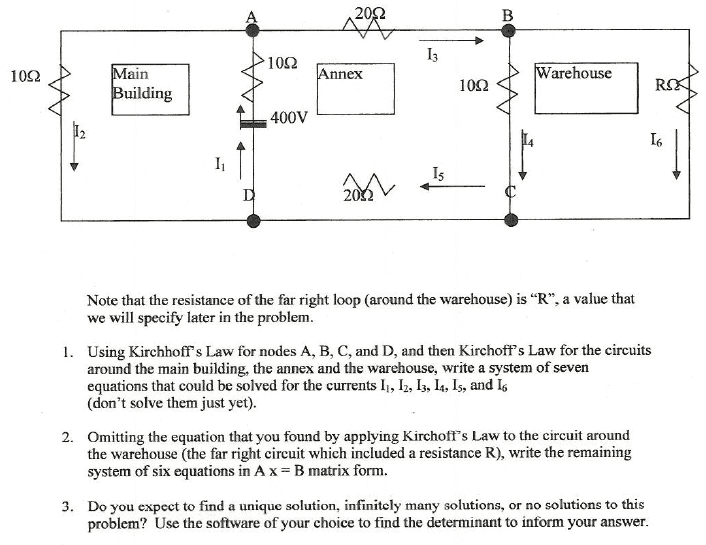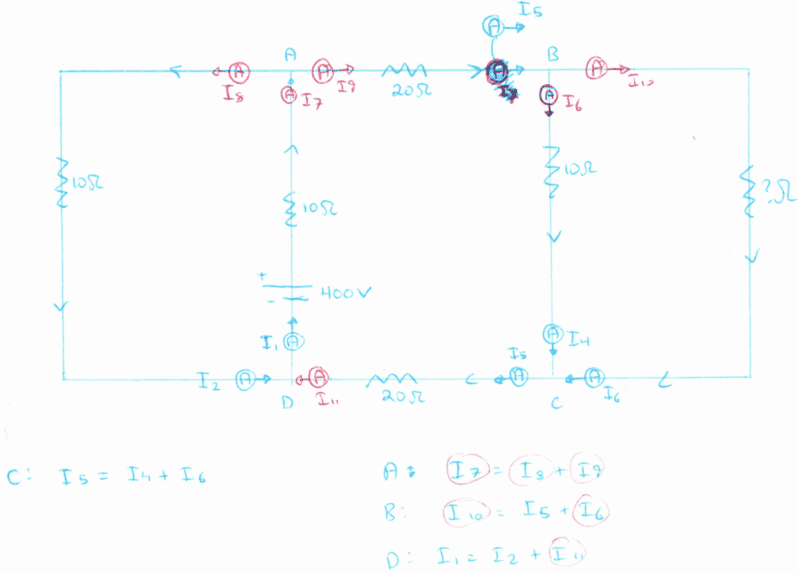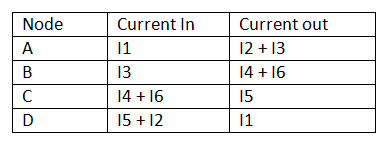# Circuits, Kirchoff's Law for Nodes

## Homework Statement

Hello, This is part of a set of questions I need to do for a project
I just need help getting started. I've been looking at this forever and I can't figure it out.## Homework Equations

Kirchhoff's Law for Nodes:
Current going into a node = current going out of a node

Kirchhoff's Voltage Law: In one traversal of any closed loop, the sum of voltage rises = the sum of voltage drops.

## The Attempt at a Solution

I really have no idea what I'm doing, but I redrew the figure to make it more clear for myself. Please, I need any sort of help. I really don't understand how to get my equations right, I believe after I have my equations down it'll be smooth sailing from there.#### Attachments

Simon Bridge
Homework Helper
You have to pay attention to the directions of the arrows you have drawn.
For the nodes, it usually does not matter which direction you draw the arrows, though it helps to have at least one arrow going in and make sure you follow the currents around since current out of a component = current into a component.

Take a look at what you've drawn for node A.
On your diagram, I8 and i9 go out while I7 is in, so you write
I7=I8+I9 ... correctly.

Follow I8 around the circuit - see that I2 = I8? For similar reasons, I1 = I7 and so on - which is why the diagram that comes with the problem has so few currents on it.

So - use the current labelled on the diagram you are given.

When you get to the voltage law - you have six loops total, though you can probably get away with the obvious three. Draw arrows for each power source (batteries here) pointing from negative to positive and label with the voltage of each (there's only one here and it's done for you) but also draw arrows on all the resistors - these arrows point opposite the currents and label them IR (with the right numbers/subscripts for the I and the R).

Start at a node, and trace around one loop. Each time you go against an arrow, you subtract it, each time you go along an arrow you add it, when you get back to the start you write "=0".

This all will give you a set of simultaneous equations.

Simon Bridge, Thank you for your response. I have one fundamental question. If you say that I2 = I8, does that mean current does not drop after passing a resistor, but instead, drops when passing a node?

I will work on this problem as you have described and post what I've done soon =)

According to what you said, I managed to get the following (all though unsure if it is correct)I am having trouble finding the voltage drops

If E = IR, and we are given only E and R, we can calculate I.
How do we get a voltage drop in this case? IR will always equal E if I solve it this way.

#### Attachments

Simon Bridge
Homework Helper
Simon Bridge, Thank you for your response. I have one fundamental question. If you say that I2 = I8, does that mean current does not drop after passing a resistor, but instead, drops when passing a node?
No to both.

If you think about it - if the current did drop after a resistor, that would mean lots of electrons enter the resister and few leave. Where did they all go? Does the resistor get more and more negatively charged?

The resister is like a rough patch on the road - it is harder for the power supply to haul the electrons over that bit. But they come out at the same rate they went in.

At a node, the electrons come to a branch in the flow: some go one way and some go the another. If ten trucks arrive at a cross intersection, and you see 3 go left and 3 go right, then the other 4 must have gone right through. (If they parked, you'd end up with a truck jam, and if you saw more than ten leave why then: where did they come from?)

Simon Bridge
Homework Helper
I am having trouble finding the voltage drops

If E = IR, and we are given only E and R, we can calculate I.
How do we get a voltage drop in this case? IR will always equal E if I solve it this way.
But that is exactly what the voltage drop is!

Look back on the diagram (top). Take the left-most loop and walk it from A, anticlockwise around to C and then back to A again... this gives:

$$-10I_2 + 400 -10I_1 = 0$$

Can you see why?
The arrows on the resistors point against the current because that is the direction of increasing voltage. We just list the positive and negative voltages we encounter on our walk on one line then finish it with "= 0".

The way we are going, the first thing we hit is the left-most 10 Ohm resister, it's arrow opposes us so the effect is negative, the current through the resister is I2 so the volt drop is -10I2. The next thing is the battery/thing - we are walking in the direction of it's arrow so it's positive at +400V. Then there's another 10 Ohm resister, opposing us, with a current of I1 through it. That completes one loop, so I just put "= 0" on the end of the list.

Now you do it for the others.

also,
Thank you for explaining current to me =)
I have a question about it
at a node, does current get equally split?

if there are 10 trucks going in, do 5 of them have to go left and 5 go right. or can 1 go left and 9 go right?

Simon Bridge
Homework Helper
I have to sleep sometime you know?!

Lets see - in reverse order:

if there are 10 trucks going in, do 5 of them have to go left and 5 go right. or can 1 go left and 9 go right?

How many trucks go in which direction depends on drivers and how difficult the path is. In terms of electrical currents, the exact current that ends up going in each direction depends on the resistances around the different paths - you get more current through the least resistance.

Is my equations for the current going through the nodes correct?
Dunno, I haven't checked. Remember, I'm not supposed to do your homework for you. When you understand what you are doing you'll be able to tell anyway.

also, I'm a bit confused on one of the loops.
You think there is only one voltage drop in loop 3? How can we check this? Lets walk around the loop - start at node B and go anti-clockwise (it's my favorite direction as I'm very sinister).

From B-C we encounter a 10 Ohm resistor whose arrow points against us (you forgot to draw the arrows on the resistors - they always point against the current) so that you get a factor of -10I4 from this.

From C-B round the long way we come to another, unknown, resistor whose arrow is with us so this adds a factor of +RI6 after which we end up back where we started. So we put an "= 0" on the end to give:

-10I4 +RI6 = 0

How many voltages was that? I make it two!

With three loops and four nodes you should have seven equations. The six currents and R make seven unknowns - so you have a system of simultaneous equations. Have fun.

You will probably find a current that is negative - this just means the arrow was drawn the wrong way around.

SimonBridge, thank you sooo much for helping me with this. I think I understand. =)